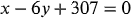1
81
views
15c
Problem

Problem 15c

Chapter 1: Functions and ModelsTextbook ExpertVerified Tutor
21 Oct 2021

Given information

According to the question we have to take the chirps per minute(N) on the x-axis and temperature T on the y-axis.

Given,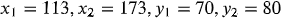also,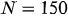Step-by-step explanation

Step 1.

The formula for the linear equation: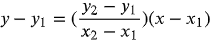Plugging the values,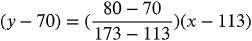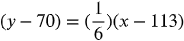On evaluating,